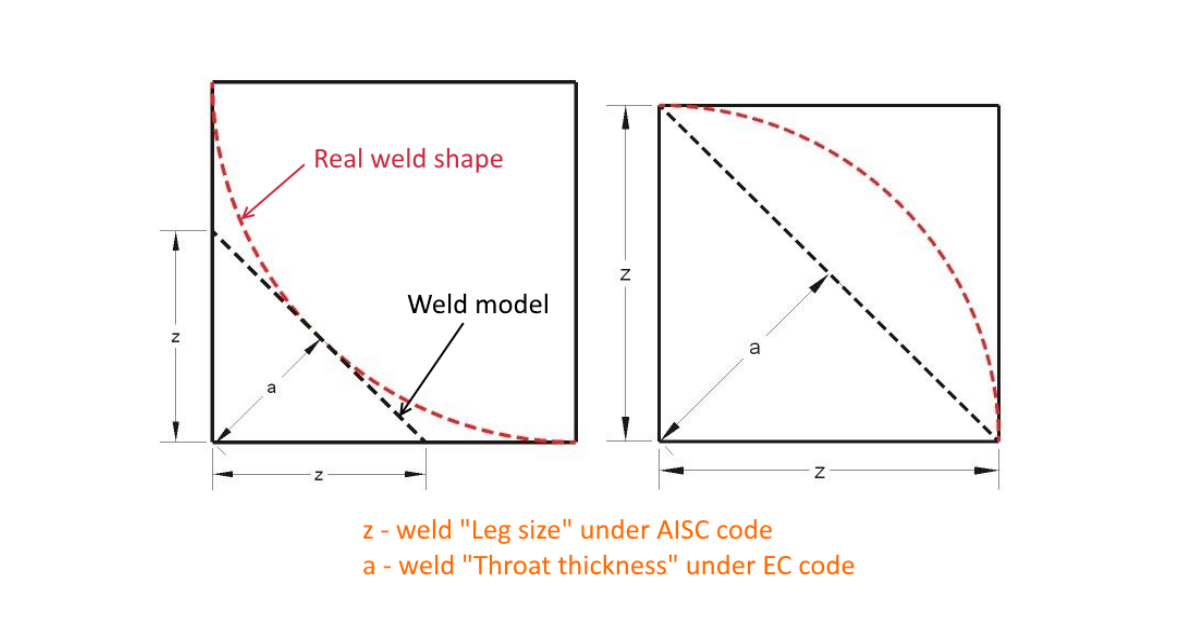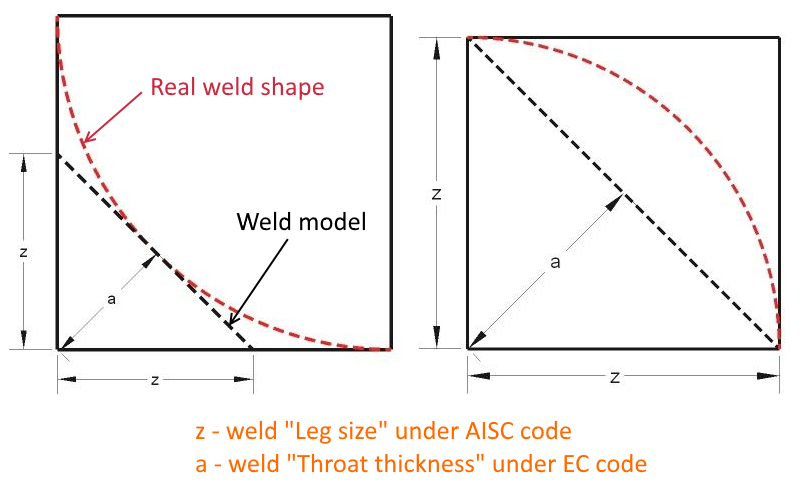### Choose language# Weld size differences between EC and AISC (CISC) codes

$$Under EN, the weld size is defined as the parameter a, which is the weld throat thickness.Under AISC (CISC), the weld size is defined as the parameter z, which is the weld leg size.You can simply calculate a from z and vice versa using the Pythagorean Theorem.$$